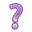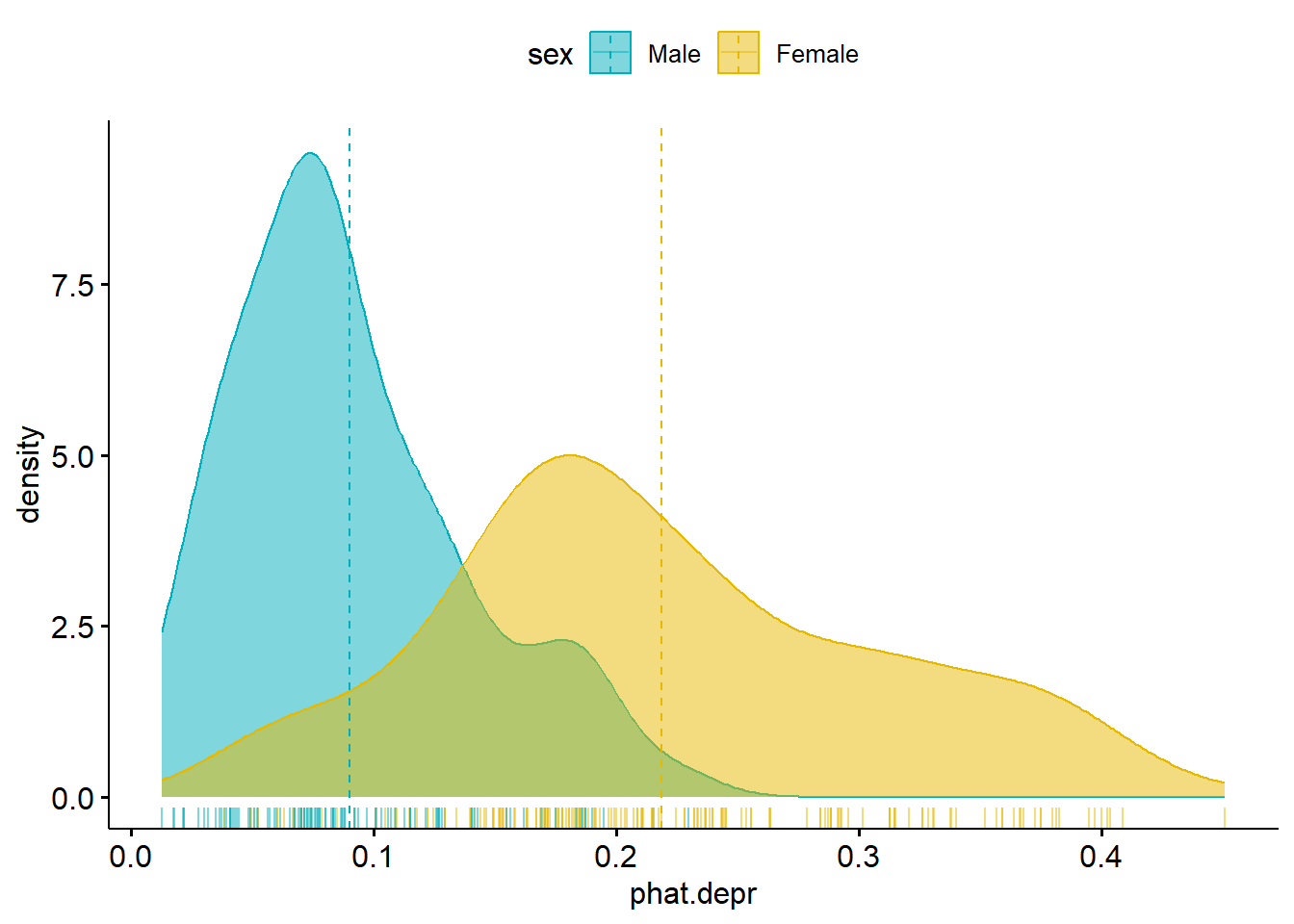## 11.1 Calculating predictions

So what if you want to get the model predicted probability of the event for all individuals in the data set? There’s no way I’m doing that calculation for every person in the data set.

Using the main effects model from above, stored in the object mvmodel, we can call the predict() command to generate a vector of predictions for each row used in the model.

Any row with missing data on any variable used in the model will NOT get a predicted value.

The mvmodel object contains a lot of information. I recommend you look at str(mvmodel) on your own time as it’s too much to print out here. The important pieces for this section is that the data used in the model (all complete case records) are stored.

mvmodel <- glm(cases ~ age + income + sex, data=depress, family="binomial")
model.pred.prob <- predict(mvmodel, type='response')

Calling dim(model.pred.prob) gives us 294. This matches the number of complete case records used to build the model. This is the same length as mvmodel$y, so we can bind them together in a data frame (useful for plotting). The model.pred.prob is a vector of individual predicted probabilities of the outcome (being depressed). To classify individual $$i$$ as being depressed or not, we draw a binary value ($$x_{i} = 0 or 1$$), with probability $$p_{i}$$ by using the rbinom function, with a size=1. set.seed(12345) #reminder: change the combo on my luggage plot.mpp <- data.frame(pred.prob = model.pred.prob, pred.class = rbinom(n=length(model.pred.prob), size=1, p=model.pred.prob), truth = mvmodel$y)
##    pred.prob pred.class truth
## 1 0.21108906          0     0
## 2 0.08014012          0     0
## 3 0.15266203          0     0
## 4 0.24527840          1     0
## 5 0.15208679          0     0
## 6 0.17056409          0     0

Applying class labels and creating a cross table of predicted vs truth:

plot.mpp <- plot.mpp %>%
mutate(pred.class = factor(pred.class, labels=c("Not Depressed", "Depressed")),
truth = factor(truth, labels=c("Not Depressed", "Depressed")))

table(plot.mpp$pred.class, plot.mpp$truth)
##
##                 Not Depressed Depressed
##   Not Depressed           195        35
##   Depressed                49        15

The model correctly identified 195 individuals as not depressed and 15 as depressed. The model got it wrong 49 + 25 times.Is this good? What if death were the event?

#### 11.1.0.1 Distribution of Predictions

Another important feature to look at is to see how well the model discriminates between the two groups in terms of predicted probabilities. Let’s look at a plot:

ggplot(plot.mpp, aes(x=truth, y=pred.prob, fill=truth)) +
geom_jitter(width=.2) + geom_violin(alpha=.4) + theme_bw()• What do you notice in this plot?
• What can you infer?